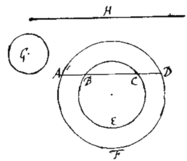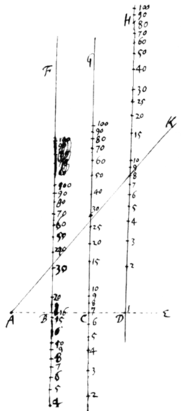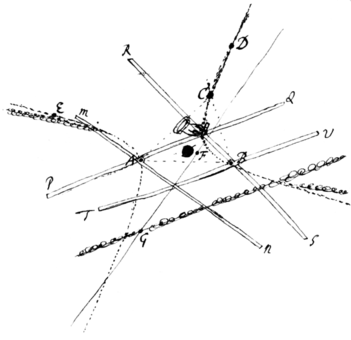<1r>

Cambridge Aug 20 1672.

Sr

Since yor last I have tryed the calculation for finding by an infinite series the content of ye second segments of an Ellipsoid. The first series yt I met with was this $\begin{array}{ccccccccc}\begin{array}{r}\phantom{\frac{b}{0}}2rb\\ -\frac{{b}^{3}}{3r}\\ -\frac{{b}^{5}}{20{r}^{3}}\\ -\frac{{b}^{7}}{56{r}^{5}}\\ \text{&c}\end{array}\right\}& a\phantom{\frac{b}{0}}& \begin{array}{r}\phantom{\frac{0}{0}}-\frac{b}{3r}\\ -\frac{{b}^{3}}{18{r}^{3}}\\ -\frac{{b}^{5}}{40{r}^{5}}\\ \text{&c}\end{array}\right\}& {a}^{3}\phantom{\frac{0}{0}}& \begin{array}{r}\phantom{\frac{b}{0}}-\frac{b}{20{r}^{3}}\\ -\frac{{b}^{3}}{40{r}^{5}}\\ \text{&c}\end{array}\right\}& {a}^{5}\phantom{\frac{0}{0}}& \begin{array}{r}\phantom{\frac{b}{0}}-\frac{b}{56{r}^{5}}\\ \text{&c}\end{array}& {a}^{7}\phantom{\frac{0}{0}}& \begin{array}{r}\phantom{\frac{b}{0}}\text{&c.}\end{array}\end{array}$ Which upon comparison proved ye same wth Mr Gregories & therefore I have exprest it in ye same letters. I tryed two or thre others, but could fine {sic} none more simple. Wherefore since I understand your designe is to get a rule for guageing vessells, I|t|his Problem having so bad success for yt end I shall in its stead present you wth this following expedient.

Let AFDA represent the vessell viewed endwiseits perimeter at the middle being AFD, |&| at ye end BEC, & ye top of ye liquor ABCD. Also let G be a circle whose semidiameter is equall y|t|o ye difference of ye semidiameters of ye other two circles AFD & BEC, & H the whole length of ye vessel. Find by a table or instrument composed for that purpose, the segments AFD, & BEC, & the w{illeg}|h|ole circle G; & $\frac{2}{3}AFD+\frac{1}{3}BEC-\frac{1}{15}GinH$ shall be the whole quantity of the liquor {illeg} in ye vessel. This rule is not exact but approaches the content of ye Parabolick spindle exactly enough for practice when the {illeg}top of ye liquor buts upon ye end of ye vessell. If ye vessel be just half full tis exact; if more then half full, tis something to little; if lesse yn half full, too much.

The approximating ye roots of affected Æquations by Gunters line is thus. Let ye æquation for instance be ${x}^{3}-7xx+16x-12=0$: to resolve wchplace thre of Gunters {illeg}|R|ulers BF, CG, & DH parallel & equally distant from one another, & to any line AE wch crosseth then all apply ye number 1 of the |t|f|h|ir{s}t|d| {illeg}|R|uler DH, ye number 7 of ye second, CG, & ye number 16 of ye first BF, accordingly as ye coefficients of ye æquation are. Then in ye cross line taking ye point A as far from B as B is from C apply any ruler to that point A, about wch whilst you turne it slowly observe the numbers where it cuts the other rulers untill you see ye summ of ye other numbers on the first & third ruler equall to ye \sum of ye/ resolvend 12 & the number on ye other ruler, wch when it happens the number on ye 3d ruler shall be ye cube of ye desired root. Thus in this case you see $8+32=12+28$, & therefore 8 the number on ye 3d ruler is ye cube of ye desired root wch consequently is 2.

The application of this to any æquations of higher di <1v> mensions is obvious. As a{illeg}|ls|o so to proportion ye rulers BF, CG, DH, &c yt ye line AK may be carried over them with parrallel motion.

The description of a Conick section wch shall pass through five given points is this. Let the five points be A, B, C, D, & E any three of wch joyn to as A, B, & C joyn to make ye a rectilinear triangle ABC, to any two angles of wch \as A & B/ apply {illeg} two sectors, their poles to ye angular points, & their leggs to the sides of ye triangle. And so dispose themthat they may turne freely abouth their poles A & B without varying the angles they are thus set at. Which done, apply to ye other two points D & E successively their two leggs \PQ & RS/ wch were before applyed to C (wch leggs for distinction sake may be called their describing leggs & t{illeg}|he| other two \MN & TV/ wch were applyed to AB, their describing \directing/ leggs,) & marke the intersections of their directing leggs, wch intersections suppose to be F when ye application was made to D, & G when made to E. Draw the right line FG & produce it infinitely both ways. And then if you move the rulers in such manner that their directing leggs doe continually intersect one another at the line GF, the intersection of their other leggs shall describe the conic section wch will pass through all the said five given points. {illeg}

If three of the given points lye {illeg} in the same streight line tis impossible for any conick section to pass through them all, And in that case you shall have instead {illeg} \thereof/ two streight lines.

Much after the same manner a Con. Sect. may be described wch shall pass through 4 given points & touch a given line, or pass through 3 given points & toug|c|h two given lines, whether those lines be right or curved. &c

I presume it will not be an unpleasing speculation to yor Mathematicians to find out ye Demonstration of this Theorem. As also to determin the center{s}{}, diameters, axes, vertices, & Asymptotes of ye Con. sect. thus described or to describe a Parabola wch shall pass through 4 given points. And there I omit them.

I herewith send you a set of Problems for construing æquations wch that I might not forget them I heretofore set down rudely as you'le find. And therefore I think 'em not fit to be seen by any but yor selfe, wch therefore you may return when you have perused them. How ye afforesaid descriptions are to be applyed to thof ye Conick sections are to be applyed to these constructions I need not tell you.

Borellius, of whom you desire my opinion, I esteem among the middle sort of Authors. I find not that he hath added any thing considerable to ye science{s} of motion but onely proved things already evidently known. Nor hath he done yt wthout some Paralogisms, as in ye proofs of ye 207|9|th, 212th, & 260th Propositio{ns.} And some of them are not onely proved parallogistically but are also fals as <2r> the 233d & those yt depend on it, but yet he may be of good i|u|se to young students in Mechanicks.

Sr I shall trouble you no further at prsent, but subscribe my self

yor very humble servant
I. Newton
<2v>

These to Mr John Collins

|Mr Newton about the Logmes Rulers and Mouvable angles|

|From No. 4 of the selected papers.|